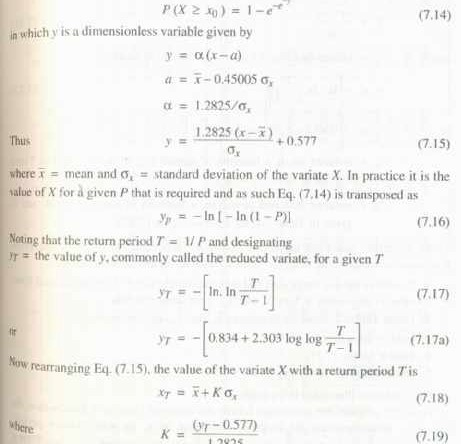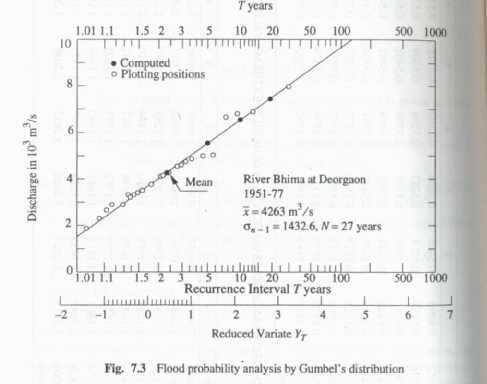Categories

# GUMBEL’S METHOD

This extreme value distribution was introduced by Gumbel (1941) and is commonly  known as gumbel’s  distribution.

It  is  one  of  the  most  widely  used probability-distribution  functions for extreme values in hydrologic and meteorologic studies for  prediction of flood peaks, maximum rainfalls, maximum wind speed, etc.

Gumbel defined  a flood as the largest of the 365 daily flows and the annual series  flood  flows constitute  a series of largest  values  of flows.

According  to his theory extreme events, the probability of occurrence of an event equal to or larger than a value x0 is

Since the practical annual data series of extreme events such as floods., maximum rainfall depths etc., all have finite lengths of record, Eq. (7.19) is modified to account for finiite N as given below for practical use.## Gumbel Probability Paper

The  Gumbel  probability  paper  is  an  aid  for  convenient  graphical representation of Gumbel’ s distribution.

It consists of an abscissa specially marked for various  convenient values of the return period T. To construct the T scale on the abscjssa.

First construct an arithmetic scale of YT values, say from  —2 to + 7, as in Fig. 7.3. For selected values of T, say 2, 10, 50, 100,500 and 1000, find the values of YT by Equation (7.22) and mark off those positions on the abscissa. The T —scale is now ready for use (Fig. 7.3)logarithmic scale. Since by Eqs (7.18) and (7.19) x varies linearly with yr, a Gumbel distribution will plot as a straight line on a Gumbel probability paper. This property can be used advantageously for graphical extrapolation, wherever necessary.Ramazan Saracisays: